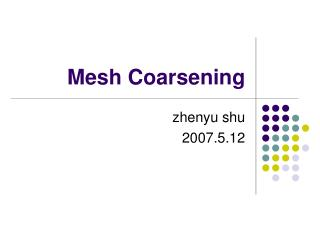DownloadDownload PresentationMesh Coarsening

Mesh Coarsening

Télécharger la présentationMesh Coarsening

- - - - - - - - - - - - - - - - - - - - - - - - - - - E N D - - - - - - - - - - - - - - - - - - - - - - - - - - -
Presentation Transcript

1. Mesh Coarsening zhenyu shu 2007.5.12

2. Mesh Coarsening • Large meshes are commonly used in numerous application area • Modern range scanning devices are used • High resolution mesh model need more time and more space to handle • Large meshes need simplification to improve speed and reduce memory storage

3. Mesh Coarsening • Size, quality and speed • Mesh optimization • Many simplification methods now

4. QEM • Garland M, Heckbert P. Surface simplification using quadric error metrics. In: Proceedings of the Computer Graphics, Annual Conference Series. Los Angeles: ACM Press, 1997. 209~216

5. QEM • Quadric Error Metric method • Using Pair Contraction to simplify the mesh • Minimize Quadric function when contracting • Define Quadric

7. Pair Contraction

8. Pair Selection • Condition • is an edge or • , where t is a threshold • When performing , • Choose position of minimizing • If A is not invertible, choose among two endpoints and midpoint of two endpoints

9. Algorithm Summary • Compute the Q matrices for all the initial vertices. • Select all valid pairs. • Compute the optimal contraction target for each valid pair • Place all the pairs in a heap keyed on cost with the minimumcost pair at the top. • Iteratively remove the pair of least cost from the heap, contract this pair, and update the costs of all valid pairs involving v1.

10. Advantage • Efficiency, local, extremely fast • Quality, maintain high fidelity to the original mesh • Generality, can join unconnected regions of original mesh together

11. Result Original model An approximation with 69451 triangles with 1000 triangles

12. Topology manipulation • Hattangady N V. A fast, topology manipulation algorithm for compaction of mesh/faceted models[J]. Computer-Aided Design. 1998, 30(10): 835-843.

13. Edge collapsing

14. Edge swapping

15. Edge smoothing • let N be the average of all Ci

16. Data Structure of mesh model • A type of data structure to present mesh model for reference

17. Remeshing • Surazhsky V, Gotsman C. Explicit surface remeshing[C]. Aachen, Germany: Eurographics Association, 2003 • Improve mesh quality by a series of local modification of the mesh geometry and connectivity

18. Vertex Relocation • with neighbors • Find new location of to satisfy some constraints, e.g. improving the angles of the triangles incident on

19. Vertex Relocation • Map these vertices into a plane, is mapped to the origin, satisfy • The angles of all triangles at are proportional to the corresponding angles and sum to

20. Vertex Relocation • Let new position of be the average of to improve the angles of the adjacent faces • Bring new position of back to the original surface by maintain same barycentric coordinate

21. Detail • (c) is original mesh, (b) is new mesh, (d) is 2D mesh which defines a parameterization of (c) • Use the same barycentric coordinates in (a) and (d)

22. Area-based Remeshing • Area equalization is done iteratively by relocating every vertex such that the areas of the triangles incident on the vertex are as equal as possible • Extending method above to relocating vertices such that the ratios between the areas are as close as possible to some specified values

23. Area-based Remeshing • Here is the area of triangle , is the area of polygon

24. Area-based Remeshing

25. Curvature sensitive remeshing • More curved region contain small triangles and a dense vertex sampling, while almost flat regions have large triangles • Define density function as here K and H are approximated discrete Gaussian and mean curvatures Meyer M, Desbrun M, Schroder P, et al. Discrete differential geometry operator for triangulated 2-manifolds [A]. In: Proceedings of Visual Mathematics'02, Berlin, 2002. 35～57

26. Result

27. Result

28. CVD • Valette S, Chassery J M. Approximated Centroidal Voronoi Diagrams for Uniform Polygonal Mesh Coarsening[J]. Computer Graphics Forum. 2004, 23(3): 381-389

29. Voronoi Diagram • Given an open set of Rm, and n different points zi; i=0,...,n-1, the Voronoi Diagram can be defined as n different regions Visuch that: where d is a function of distance.

30. Centroidal Voronoi Diagram • A Centroidal Voronoi Diagram is a Voronoi Diagram where each Voronoi site zi is also the mass centroid of its Voronoi Region: here is a density function of

31. Centroidal Voronoi Diagram • Centroidal Voronoi Diagrams minimize the Energy given as: • On mesh, Energy above becomes to

32. Construct CVD • Here • Construct CVD based on global minimization of the Energy term E2

33. Algorithm Summary • Randomly choose n different cells in mesh and these cells form n regions • Cluster all cells in mesh by extending these regions and choosing correct cells’ owner to minimize the energy term E2 • Now calculate each center of these regions and replace each region with it’s center • Triangulate and get new mesh

34. Clustering

35. Triangulate

36. Sample

37. Sample

38. Result Quality and Speed

39. Pros and Cons • Pros • High quality of result • Optimization of original mesh • Cons • Slow • Global

40. Thanks